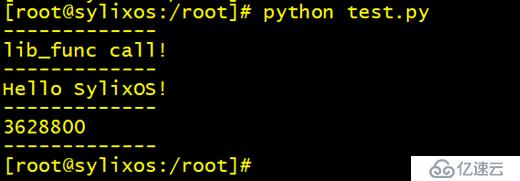# 关于我们

< 返回

## 2 SylixOS Python简介

Python是一门面向对象的解释型的脚本语言，Python具有丰富和强大的库。它常被昵称为胶水语言，能够把用其他语言制作的各种模块（尤其是C/C++）很轻松地联结在一起。常见的一种应用情形是，使用Python快速生成程序的原型（有时甚至是程序的最终界面），然后对其中有特别要求的部分，用更合适的语言改写，比如3D游戏中的图形渲染模块，性能要求特别高，就可以用C/C++重写，而后封装为Python可以调用的扩展类库。

## 3 SylixOS Python扩展库开发

### 3.1 基于python ctypes模块开发

SylixOS下的Python支持ctypes内建模块。此模块的CDLL方法会调用SylixOS操作系统的dlopen()函数，从而导入用户开发的C/C++动态库。

#include <stdio.h>

void lib_func (void)

{

printf("lib_func call!\n");

}

void hello (void)

{

printf("Hello SylixOS!\n");

}

int fact(int n)

{

if (n <= 1)

return 1;

else

return n * fact(n - 1);

}

lib_demo编译上传至目标设备中。新建一个Python脚本test.py，使用VI编辑器编辑python脚本，内容如下：

import ctypes

demo=ctypes.CDLL("/lib/lib_demo.so")

print("-------------")

demo.lib_func()

print("-------------")

demo.hello()

print("-------------")

print(demo.fact(10))

print("-------------")3.1 python脚本执行结果

### 3.2 基于Python扩展库模板开发

3.2 选择动态库头文件路径

a、包含Python头文件；

b、利用样板来包装函数；

lib_demo中的fact()函数封装如下（并非修改原函数，而是添加封装函数）

static PyObject *lib_demo_fact(PyObject *self,PyObject *args) {

int num;

if(!(PyArg_ParseTuple(args,"i",&num))) {       //获取Python传递的参数

return NULL;

}

return (PyObject*)Py_BuildValue("i",fact(num));

//将结果转换为Python类型并返回

}

c、为每个模块增加PyMethodDef ModuleMethods[]数组；

static PyMethodDef lib_demoMethods [] = {

{"fact",lib_demo_fact,METH_VARARGS},            //函数名，包装函数名，解析

{"hello",lib_demo_hello,METH_NOARGS},

{"lib_func",lib_demo_lib_func,METH_NOARGS},

{NULL,NULL},                                         //作为结束

};

d、编写初始化函数。

static struct PyModuleDef lib_demoModule = {

"lib_demo",                  //模块名

NULL,

-1,

lib_demoMethods             //上面的数组

};

PyMODINIT_FUNC

PyInit_lib_demo(void)

{

return  PyModule_Create(&lib_demoModule);

}

#include <Python.h>

#include <stdio.h>

int lib_func (void)

{

printf("lib_func call!\n");

return 0;

}

int hello (void)

{

printf("Hello SylixOS!\n");

return 0;

}

int fact(int n)

{

if (n <= 1)

return 1;

else

return n * fact(n - 1);

}

static PyObject *lib_demo_fact(PyObject *self,PyObject *args) {

int num;

if(!(PyArg_ParseTuple(args,"i",&num))) {                  //获取Python传递的参数

return NULL;

}

return (PyObject*)Py_BuildValue("i",fact(num));           //将结果转换为Python类型并返回

}

static PyObject *lib_demo_hello(PyObject *self,PyObject *args) {

return (PyObject*)Py_BuildValue("i",hello());

}

static PyObject *lib_demo_lib_func(PyObject *self,PyObject *args) {

return (PyObject*)Py_BuildValue("i",lib_func());

}

static PyMethodDef lib_demoMethods[] = {

{"fact",lib_demo_fact,METH_VARARGS},//函数名，包装函数名，解析

{"hello",lib_demo_hello,METH_NOARGS},

{"lib_func",lib_demo_lib_func,METH_NOARGS},

{NULL,NULL},//作为结束

};

static struct PyModuleDef lib_demoModule = {

"lib_demo",                //模块名

NULL,

-1,

lib_demoMethods            //上面的数组

};

PyMODINIT_FUNC

PyInit_lib_demo(void)

{

return  PyModule_Create(&lib_demoModule);

}

import lib_demo as demo

print("-------------")

demo.lib_func()

print("-------------")

demo.hello()

print("-------------")

print(demo.fact(10))

print("-------------")3.3 test2脚本执行结果

/template/Home/Dawn/PC/Static